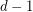# hypergraph

## A generalization of Vizing's Theorem? ★★

Author(s): Rosenfeld

Conjecture   Letbe a simple-uniform hypergraph, and assume that every set ofpoints is contained in at mostedges. Then there exists an-edge-coloring so that any two edges which sharevertices have distinct colors.

Keywords: edge-coloring; hypergraph; Vizing

## Ryser's conjecture ★★★

Author(s): Ryser

Conjecture   Letbe an-uniform-partite hypergraph. Ifis the maximum number of pairwise disjoint edges in, andis the size of the smallest set of vertices which meets every edge, then.

Keywords: hypergraph; matching; packing